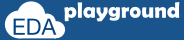## Argument Passing

SystemVerilog provides below means for passing arguments to functions and tasks,

• argument pass by value
• argument pass by reference
• argument pass by name
• argument pass by position

also, functions and tasks can have default argument values.

## argument pass by value

In argument pass by value,
the argument passing mechanism works by copying each argument into the subroutine area.
if any changes to arguments within the subroutine, those changes will not be visible outside the subroutine.

### argument pass by value example

Variables x and y are passed as an argument in the function call sum, changes to the argument x within the function is not visible outside.

```module argument_passing;
int x,y,z;
```
```  //function to add two integer numbers.
function int sum(int x,y);
x = x+y;
return x+y;
endfunction

initial begin
x = 20;
y = 30;
z = sum(x,y);
\$display("-----------------------------------------------------------------");
\$display("\tValue of x = %0d",x);
\$display("\tValue of y = %0d",y);
\$display("\tValue of z = %0d",z);
\$display("-----------------------------------------------------------------");
end
endmodule```

Simulator Output
-----------------------------------------------------------------
Value of x = 20
Value of y = 30
Value of z = 80
-----------------------------------------------------------------
Execute the above code on## argument pass by reference

In pass by reference, a reference to the original argument is passed to the subroutine.

As the argument within a subroutine is pointing to an original argument, any changes to the argument within subroutine will be visible outside.

To indicate argument pass by reference, the argument declaration is preceded by keyword ref.

Any modifications to the argument value in a pass by reference can be avoided by using const keyword before ref, any attempt in changing the argument value in subroutine will lead to a compilation error.

### argument pass by reference example

variables x and y are passed as an argument in the function call sum, changes to the argument x within the function, is visible outside.

```module argument_passing;
int x,y,z;

//function to add two integer numbers.
function int sum(ref int x,y);
x = x+y;
return x+y;
endfunction

initial begin
x = 20;
y = 30;
z = sum(x,y);
\$display("-----------------------------------------------------------------");
\$display("\tValue of x = %0d",x);
\$display("\tValue of y = %0d",y);
\$display("\tValue of z = %0d",z);
\$display("-----------------------------------------------------------------");
end
endmodule```

Simulator Output
-----------------------------------------------------------------
Value of x = 50
Value of y = 30
Value of z = 80
-----------------------------------------------------------------
Execute the above code onAny modifications to the argument value in a pass by reference can be avoided by using const keyword before ref, any attempt in changing the argument value in subroutine will lead to a compilation error.

## argument pass by reference with the const keyword

variables x and y are passed as an argument in the function call sum, as arguments are mentioned as const, changes to the argument x within the function leads to a compilation error.
```module argument_passing;
int x,y,z;
```
```  //function to add two integer numbers.
function int sum(const ref int x,y);
x = x+y;
return x+y;
endfunction

initial begin
x = 20;
y = 30;
z = sum(x,y);
\$display("-----------------------------------------------------------------");
\$display("\tValue of x = %0d",x);
\$display("\tValue of y = %0d",y);
\$display("\tValue of z = %0d",z);
\$display("-----------------------------------------------------------------");
end
endmodule```

Simulator Output
'const' variable is either driven or connected to a non-const variable.
Variable 'x' declared as 'const' cannot be used in this context
Source info: x = (x + y);

1 error
Execute the above code on## default argument values

The default value can be specified to the arguments of the subroutine.

In the subroutine call, arguments with a default value can be omitted from the call.
if any value is passed to an argument with a default value, then the new value will be considered.

### an argument with default value example

variables x, y and z of the subroutine has a default value of 1,2 and 3 respectively, in the function call value is passed only for z. x and y will take the default value.

```module argument_passing;
int q;

//function to add three integer numbers.
function int sum(int x=5,y=10,z=20);
return x+y+z;
endfunction

initial begin
q = sum( , ,10);
\$display("-----------------------------------------------------------------");
\$display("\tValue of z = %0d",q);
\$display("-----------------------------------------------------------------");
end
endmodule```

Simulator Output
-----------------------------------------------------------------
Value of x = 20
Value of y = 30
Value of z = 25
-----------------------------------------------------------------
Execute the above code on## argument pass by name

In argument pass by name, arguments can be passed in any order by specifying the name of the subroutine argument.

### argument pass by name example

value to the second argument is passed first by specifying the argument name.

```module argument_passing;
int x,y,z;

function void display(int x,string y);
\$display("\tValue of x = %0d, y = %0s",x,y);
endfunction

initial begin
display(.y("Hello World"),.x(2016));
end
endmodule```

Simulator Output

Value of x = 2016, y = Hello World